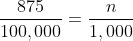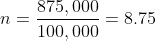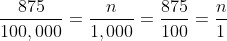### moving from n per 100,000 to n per 1,000

I thought I'd make this post because I see that students sometimes run into problems when they find data reported as n per 100,000. How to move from that to n per 1,000? It's actually rather easy.

Let's say a mortality rate is reported as 875 per 100,000. To turn it into n per 1,000, just move the decimal to the corresponding places to the left-- e.g., move it two places to the left for both numbers: 8.75 per 1,000.

Here's the long form math:cross-multiplying gives you 875,000 = 100,000nOR just notice that